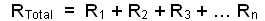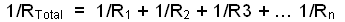Home / Electrical MCQs / DC Circuits MCQs with Answers

# DC Circuits MCQs with Answers

Want create site? Find Free WordPress Themes and plugins.
1. The minimal electrical circuit must contain three parts-a source, a path and a:

 B. battery
 C. circuit
 D. supply

2. The source of supply of an electrical circuit is:

 A. always an AC network
 B. where the energy comes from
 C. the part of the circuit where the current does not flow
 D. the section where the energy is consumed

3. The load of an electrical circuit is the part that:

 A. supplies the energy for the circuit
 B. conducts the current to the working device
 C. converts electricity into some form of work
 D. must always be connected a short circuit

4. An electrical circuit needs a conductor to:

 A. act as the circuit load
 B. provide the energy for the circuit
 C. ensure that a short circuit cannot occur
 D. carry the current to the load

5. Switches control current flow within a circuit. When a circuit is open:

 A. no current flows
 B. maximum current flows
 C. short-circuit current flow
 D. the supply is connected to the load

6.
With reference to the simple circuit shown above, the element marked ‘3’ is the:

 A. source
 C. path
 D. battery

7. For ammeters to read the current in a circuit, the ammeter must be connected so that:

 A. it is in parallel with the circuit
 B. the voltage will flow around the ammeter
 C. the current passes through the ammeter

8. In a series-connected circuit, there is only one path and the current from the source:

 A. flows around some components
 B. only flows through the source
 C. does not flow through the loads
 D. flows through each component

9.
The above drawing shows a:

 A. series circuit
 B. parallel circuit
 C. compound circuit
 D. racing circuit

10. In a parallel-connected circuit:

 A. there is only one current path
 B. there is one path for each load
 C. the loads are connected in series
 D. a supply source is not required

11.
In the diagram, the loads are connected:

 A. in a series configuration
 B. in a series/parallel combination
 C. in a parallel configuration
 D. so that the current flows through one after another

12. Compound circuits are made up of:

 A. compound supply sources only
 C. only those parts that are in parallel with the supply
 D. both series and parallel parts

13.
In the above diagram, the lamps are connected as a:

 A. compound circuit
 B. series circuit only
 C. parallel circuit only
 D. simple circuit

14.
The above diagram represents a:

 A. simple circuit
 B. complex circuit
 C. series circuit
 D. parallel circuit

15. When resistors are connected together, they can be replaced by a single resistor with the same overall resistance as the set of resistors. A resistor that has the same value as a group of combined resistors is called:

 A. a compound resistance
 B. a complex resistance
 C. an equivalent resistance
 D. an elaborate resistance

16. Loops are an engineering title for what electricians would normally refer to as:

 A. voltage nodes
 B. current nodes
 C. voltage paths
 D. current paths

17.
The number of current loops in the above circuit is:

 A. 5
 B. 4
 C. 6
 D. 3

18. The common electrical abbreviation for the potential difference between two points is:

 A. VD
 B. PD
 C. EMF
 D. DIFF

19.
With reference to the memory jogger called ‘Ohm’s Triangle’ shown above, if the value of the current in a given circuit is required it can be determined by:

 A. multiplying the voltage by the resistance
 B. multiplying the power by the voltage
 C. dividing the voltage by the resistance
 D. dividing the power by the resistance

20.
This diagram shows the power wheel which can be used as a memory jogger when solving simple circuit analysis problems. This wheel is derived from:

 A. Ohm’s Law and the current divider rule
 B. Kirchhoff’s Law and the power equation
 C. Kirchhoff’s law and Ohm’s Law
 D. Ohm’s Law and the power equation

21. Kirchhoff’s Voltage Law states that in any given circuit, the algebraic sum of the applied EMFs is equal to the:

 A. algebraic sum of the voltage drops
 B. algebraic difference between any two voltage drops
 C. value of the algebraic applied current
 D. sum of the algebraic resistance values

22. The following formula can be used to determine the total resistance in a series circuit.In the formula the symbol R1 stands for the:

 A. total circuit resistance
 B. resistance of resistor R1
 C. current through resistor R1
 D. voltage drop across resistor R1

23.
The equivalent resistance to replace the three resistors in the series circuit shown above is:

 A. 5 W
 B. 10 W
 C. 35 W
 D. 40 W

24. The equivalent resistance of a number of series resistors is always:

 A. less than any individual resistance
 B. equal to the value of any individual resistance
 C. equal to the product of the individual resistances
 D. greater than any individual resistance

25. When a number of components are in series, the current must pass through each component to return to the source. Therefore, should any one component, conductor or joint become an open circuit, the current:

 A. cannot flow and the whole circuit will fail
 B. will flow through all components but the faulty one
 C. will only flow through the open circuited joint
 D. will flow through the faulty component but not the joint

26. The voltage across any number of components connected in parallel will:

 A. be greater than the supply voltage
 B. always be the same
 C. equal to the sum, of the voltages across each component
 D. always be equal to 230 V

27. Kirchhoff’s Current Law states that the sum of the currents entering a junction:

 A. is greater than the sum of the currents leaving that junction
 B. is less than the sum of the currents leaving that junction
 C. equals the sum of the currents leaving that junction
 D. equals the supply voltage around the junction

28. The following formula can be used to determine the equivalent resistance of a number of resistors in parallel:In the formula the symbol ‘RTotal‘ stands for the:

 A. total resistance of R1 plus R2 only
 B. total supply voltage for the circuit
 C. total real current flowing through each resistor
 D. equivalent circuit resistance

29.
The equivalent resistance to replace the three resistors in the parallel circuit shown above is:

 A. 15 W
 B. 30 W
 C. 60 W
 D. 150 W

30. The equivalent resistance of a number of parallel resistors is always:

 A. greater than any individual resistance
 B. smaller than any individual resistance
 C. equal than any individual resistance
 D. equal to the sum of the individual resistance

31. In a parallel circuit, if one branch becomes open circuit, the:

 A. total current is increased
 B. total current falls to zero
 C. other branches are not affected
 D. other branches will draw more current

32. When identifying sections of a compound circuit, components that are joined by a single conductor with no other connections, are connected in:

 A. delta configuration
 B. series/parallel
 C. parallel
 D. series

33. When simplifying compound circuits by the equivalent resistance method, wherever two or more resistors are found to be in series, they may be simplified by:

 B. subtracted from each other
 C. multiplication
 D. division

34. One method that can be used to find the total power in a circuit is to find the individual power used in every component and then:

 A. apply Ohms Law to find the total power
 B. add the values to find the total power
 C. apply Kirchhoff’s Law to find the total power
 D. add the values of voltage and current to find the total power

35.
The diagram shown above has a battery of four 1.5 volt cells, connected via a switch to a 3 watt lamp. When the switch is closed, the current drawn from the battery will be:

 A. 2.0 A
 B. 1.5 A
 C. 0.5 A
 D. 0.25 A

36.
The reading on the ammeter in the above circuit will be:

 A. 2 A
 B. 1 A
 C. 0.5 A
 D. 0.25 A

37.
The reading on the voltmeter in the above circuit will be:

 A. 1 V
 B. 2.5 V
 C. 5 V
 D. 6 V

38. Parallel circuits have:

 A. no voltage drops across the resistances
 B. the same voltage across all branches in the circuit
 C. the same current through all branches in the circuit
 D. no current through the load resistances

39.
The equivalent resistance of the above circuit is:

 A. 36 W
 B. 12 W
 C. 4 W
 D. 2 W

40.
With reference to the above circuit, the reading on the ammeter will be:

 A. 6.0 A
 B. 2.5 A
 C. 2.0 A
 D. 1.5 A

41.
With reference to the above circuit, the current through resistor ‘R1’ will be:

 A. 0.5 A
 B. 1.0 A
 C. 1.5 A
 D. 2.0 A

42.
With reference to the above circuit, the reading on the ammeter will be:

 A. 0.5 A
 B. 1.0 A
 C. 1.5 A
 D. 2.0 A

43.
With reference to the above circuit, the reading on the voltmeter will be:

 A. 6.0 V
 B. 12 V
 C. 3.0 V
 D. 2.0 V

Did you find apk for android? You can find new Free Android Games and apps.